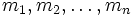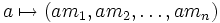# Annihilator of Noetherian module has Noetherian quotient

## Statement

### Verbal statement

Consider a Noetherian module over a commutative unital ring. The quotient of the ring by the annihilator of this module, is a Noetherian ring.

### Symbolic statement

Let$M$ be a Noetherian module over a commutative unital ring$R$. Let$I$ be the annihilator of$M$. Then the quotient ring$R/I$ is a Noetherian ring.

## Proof

Let$m_1,m_2,\ldots,m_n$ be a finite generating set for$M$. Consider a$R$-module map from$R$ to$M^n$ given by:$a \mapsto (am_1,am_2,\ldots,am_n)$

The kernel of this map is precisely$I$, so the quotient is a submodule of$M^n$.

Since$M$ is Noetherian,$M^n$ is Noetherian, and hence$R/I$ is Noetherian (as it is a submodule of a Noetherian module). But$R/I$ being Noetherian as a$R$-module is equivalent to$R/I$ being a Noetherian ring.